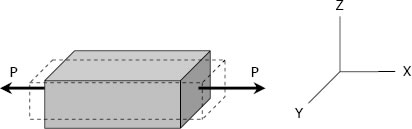# Shearing Deformation

Shearing Deformation
Shearing forces cause shearing deformation. An element subject to shear does not change in length but undergoes a change in shape.The change in angle at the corner of an original rectangular element is called the shear strain and is expressed as

$\gamma = \dfrac{\delta_s}{L}$

The ratio of the shear stress τ and the shear strain γ is called the modulus of elasticity in shear or modulus of rigidity and is denoted as G, in MPa.

$G = \dfrac{\tau}{\gamma}$

The relationship between the shearing deformation and the applied shearing force is

$\delta_s = \dfrac{VL}{A_s G} = \dfrac{\tau L}{G}$

where V is the shearing force acting over an area As.

Poisson's Ratio
When a bar is subjected to a tensile loading there is an increase in length of the bar in the direction of the applied load, but there is also a decrease in a lateral dimension perpendicular to the load. The ratio of the sidewise deformation (or strain) to the longitudinal deformation (or strain) is called the Poisson's ratio and is denoted by ν. For most steel, it lies in the range of 0.25 to 0.3, and 0.20 for concrete.$\nu = -\dfrac{\varepsilon_y}{\varepsilon_x} = -\dfrac{\varepsilon_z}{\varepsilon_x}$

where εx is strain in the x-direction and εy and εz are the strains in the perpendicular direction. The negative sign indicates a decrease in the transverse dimension when εx is positive.

Biaxial Deformation
If an element is subjected simultaneously by tensile stresses, σx and σy, in the x and y directions, the strain in the x direction is σx/E and the strain in the y direction is σy/E. Simultaneously, the stress in the y direction will produce a lateral contraction on the x direction of the amount -ν εy or -ν σy/E. The resulting strain in the x direction will be

$\varepsilon_x = \dfrac{\sigma_x}{E} - \nu \dfrac{\sigma_y}{E}$   or   $\sigma_x = \dfrac{(\varepsilon_x + \nu \varepsilon_y)E}{1 - \nu^2}$

and

$\varepsilon_y = \dfrac{\sigma_y}{E} - \nu \dfrac{\sigma_x}{E}$   or   $\sigma_y = \dfrac{(\varepsilon_y + \nu \varepsilon_x)E}{1 - \nu^2}$

Triaxial Deformation
If an element is subjected simultaneously by three mutually perpendicular normal stresses σx, σy, and σz, which are accompanied by strains εx, εy, and εz, respectively,

$\varepsilon_x = \dfrac{1}{E} [ \, \sigma_x - \nu (\sigma_y + \sigma_z) \, ]$

$\varepsilon_y = \dfrac{1}{E} [ \, \sigma_y - \nu (\sigma_x + \sigma_z) \, ]$

$\varepsilon_z = \dfrac{1}{E} [ \, \sigma_z - \nu (\sigma_x + \sigma_y) \, ]$

Tensile stresses and elongation are taken as positive. Compressive stresses and contraction are taken as negative.

Relationship Between E, G, and ν
The relationship between modulus of elasticity E, shear modulus G and Poisson's ratio ν is:

$G = \dfrac{E}{2(1 + \nu)}$

Bulk Modulus of Elasticity or Modulus of Volume Expansion, K
The bulk modulus of elasticity K is a measure of a resistance of a material to change in volume without change in shape or form. It is given as

$K = \dfrac{E}{3(1 - 2\nu)} = \dfrac{\sigma}{\Delta V/V}$

where V is the volume and ΔV is change in volume. The ratio ΔV/V is called volumetric strain and can be expressed as

$\dfrac{\Delta V}{V} = \dfrac{\sigma}{K} = \dfrac{3(1 - 2\nu)}{E}$